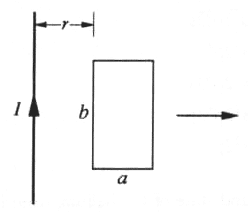GR 8677927796770177 | # Login | Register

GR9277 #55
Problem
 GREPhysics.NET Official Solution Alternate Solutions
\prob{55}A rectangular loop of wire with dimensions shown above is coplanar with a long wire carrying current I. The distance between the wire and the left side of the loop is r. The loop is pulled to the right as indicated.

What is the magnitude of the net force on the loop when the induced current is i?

Electromagnetism}Magnetic Force

The magnetic force of a wire is given by , where I is the current of the wire and l its length.

The field that produces the force on the loop is given by the long wire (see the previous problem for why). The field of that wire is given trivially by Ampere's Law to be , where r is the radial distance away from its center.

Only two wires from the loop contribute to the force, since the cross-product yields 0 force for the two horizontal components. Thus, the net force on the loop with current i with vertical components of length b is . Combine the fraction to get choice (D).Alternate Solutions
 h.fei102012-11-04 05:11:22 The force should be proportional to the length b, remember the formula F=BIL in high school, so eliminate A,B,E. The force is related to r, so only D remains.Reply to this commentjmason862009-09-06 16:13:03 lots of solutions up here already but... There's no reason that ln should come into this calculation. Eliminate (A) and (B) As other have said, as r--> F-->0. Eliminates (C) and (E)Reply to this commentemmo
2019-12-18 06:28:15
According to this solution, \"the cross-product yields 0 force for the two horizontal components,\" but is that right? The field is pointing into the page, which is orthogonal to the line of the induced current, thus there would be force on each horizontal component. This contributes nothing to the net force due to symmetry: the top and bottom horizontal portions of the induced current cancel one another out.h.fei10
2012-11-04 05:11:22
The force should be proportional to the length b, remember the formula F=BIL in high school, so eliminate A,B,E.

The force is related to r, so only D remains.pkamal
2010-10-08 09:16:55
Here is one more way of looking at this problem.
In the limit a->0, the net force on the wire must be zero, because you will have two equal and opposite forces acting on the wire. So, the only choice that goes to zero in the limit a->0 is D. So D is the correct choice:)
 neon372010-11-12 00:56:42 incorrect. Also, A and B go to zero as ln(1)=0. This works only if you eliminate A and B first.pkamal
2010-10-08 09:16:21
Here is one more way of looking at this problem.
In the limit a->0, the net force on the wire must be zero, because you will have two equal and opposite forces acting on the wire. So, the only choice that goes to zero in the limit a->0 is D. So D is the correct choice:)Fortisimo
2010-10-05 12:52:47
C, D, E should not have the natural logs; this appears to be a typojmason86
2009-09-06 16:13:03
lots of solutions up here already but...

There's no reason that ln should come into this calculation. Eliminate (A) and (B)
As other have said, as r--> F-->0. Eliminates (C) and (E)
 RusFortunat2015-10-22 18:10:05 Thank youneutrinosrule
2008-10-04 17:36:00
you can just note that the force has to be inversely proportional to the distance from the loop to the wire... this only leaves D.spacebabe47
2007-08-28 14:55:13
there is no ln in answers C,D,and Ebootstrap
2007-04-06 12:51:52
An easy way to do this without paying too much attention to the different forces is looking at the solutions as the limit of r goes to infinity. The only answer that clearly goes to zero is choice D.
 hassanctech2007-09-30 21:16:47 That isn't true. In choices a and b the ln(r/(r+a)) and ln ((r+a)/r) both go to ln(1) as r --> infinity and ln(1) = o so choices 1 and 2 also go to zero.spacebabe47
2006-11-01 15:13:59
If the area of the loop is zero, there will be no flux, and hence no induced current and no force. So, F=0 if a=0. This eliminates A, B, and C. Also, F=0 if b=0. This eliminates E. Only choice D is left.
 spacebabe472006-11-01 15:23:30 Edit: If the area of the loop is zero, there will be no flux, and hence no induced current and no force. So, F=0 if a=0. This eliminates C. Also, F=0 if b=0. This eliminates A, B, and E. Only choice D is left.senatez
2006-10-31 20:47:42
You can also consider the limiting case where the force will go to zero as r goes to zero. This will eliminate choices C and E. From here you can make an educated guess. One would expect the force to depend on both dimensions a and b. So then chose choice D.there is no ln in answers C,D,and ELaTeX syntax supported through dollar sign wrappers $, ex.,$\alpha^2_0$produces . type this... to get...$\int_0^\infty\partial\Rightarrow\ddot{x},\dot{x}\sqrt{z}\langle my \rangle\left( abacadabra \right)_{me}\vec{E}\frac{a}{b}\$